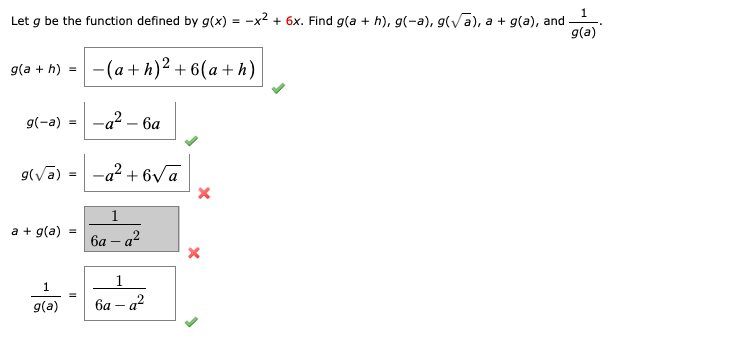# Let g be the function defined by g(x) = -x? + 6x. Find g(a + h), g(-a), g(vā), a + g(a), and - g(a) g(a + h) = -(a+ h)² + 6(a + h) g(-a) -а? — ба -a² + 6va 1 a + g(a) | 6a – a² 1 g(a) ба — а?

Questionhelp_outlineImage TranscriptioncloseLet g be the function defined by g(x) = -x? + 6x. Find g(a + h), g(-a), g(vā), a + g(a), and - g(a) g(a + h) = -(a+ h)² + 6(a + h) g(-a) -а? — ба -a² + 6va 1 a + g(a) | 6a – a² 1 g(a) ба — а? fullscreen

### Want to see this answer and more?

Experts are waiting 24/7 to provide step-by-step solutions in as fast as 30 minutes!*

*Response times vary by subject and question complexity. Median response time is 34 minutes and may be longer for new subjects.
Tagged in
MathCalculus

### Functions